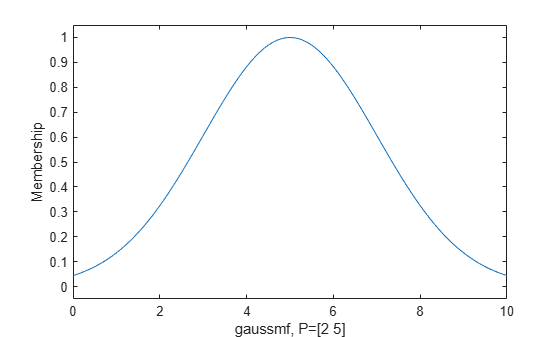gaussmf

Gaussian membership function

Description

This function computes fuzzy membership values using a Gaussian membership function. You can also compute this membership function using a fismf object. For more information, see fismf Object.

A Gaussian membership function is not the same as a Gaussian probability distribution. For example, a Gaussian membership function always has a maximum value of 1. For more information on Gaussian probability distributions, see Normal Distribution (Statistics and Machine Learning Toolbox).

example

y = gaussmf(x,params) returns fuzzy membership values computed using the following Gaussian membership function:

$f\left(x;\sigma ,c\right)={e}^{\frac{-{\left(x-c\right)}^{2}}{2{\sigma }^{2}}}$

To specify the standard deviation, σ, and mean, c, for the Gaussian function, use params.

Membership values are computed for each input value in x.

Examples

collapse all

x = 0:0.1:10;
y = gaussmf(x,[2 5]);
plot(x,y)
xlabel('gaussmf, P=[2 5]')Input Arguments

collapse all

Input values for which to compute membership values, specified as a scalar or vector.

Membership function parameters, specified as the vector [σ c], where σ is the standard deviation and c is the mean.

Output Arguments

collapse all

Membership value returned as a scalar or a vector. The dimensions of y match the dimensions of x. Each element of y is the membership value computed for the corresponding element of x.

Alternative Functionality

fismf Object

You can create and evaluate a fismf object that implements the gaussmf membership function.

mf = fismf("gaussmf",P);
Y = evalmf(mf,X);

Here, X, P, and Y correspond to the x, params, and y arguments of gaussmf, respectively.